NOTE: Each exercise is worth 10 points and can be turned in at any time before its expiration date''. At the end of the semester, I will expect you to have turned in at least 2/5 of the exercises assigned. If you do more, I will pick your best grades. If you do less, the missing grades will be counted as zeros. Altogether, these will count the same as one project.

1. (expires 2/11)     Use Maple to write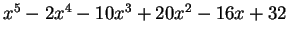as a product of exact linear factors. By exact, I mean you should leave any non-rational factors expressed as radicals; do not approximate terms like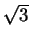as 1.73205, etc.

2. (expires 2/11)     Draw a graph showing both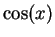and its fifth Taylor polynomial (that is,) for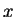between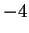and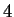. What degree of Taylor polynomial seems to be needed to get good agreement in this range'' Hint: use a variation of the command convert(taylor(cos(x),x,5),polynom) to make this work. Think of a suitable way to demonstrate that the approximation you have taken is good''- what is a good definition of good'' here?

3. (expires 2/18)    Consider the planar curve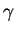defined by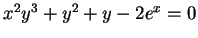. Using only Maple, find the slope of the tangent line to the curve at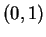. Then plot the curve and the tangent line on the same graph.
Hint: you might want to use implicitplot from the library plots. You might find implicitdiff helpful, too.

4. (expires 2/18)     Plot the function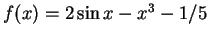, for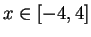. Find all the zeros of the function with an accuracy of 20 decimal digits. Hint: See Digits, fsolve.

5. (expires 2/18)     Define a Maple function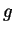that, given a positive integer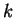yields the sum of the firstprimes. What issuch that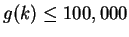but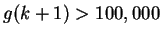? You might find sum and ithprime helpful.

6. (expires 2/18)     Use the Taylor expansion of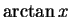near the point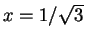to compute the value of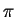to 30 places. How many terms are needed to compute the value to 50 places?

MAT 331
2002-02-10